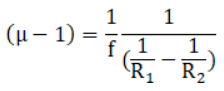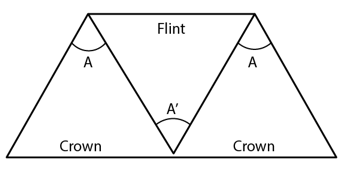# HC Verma Solutions Class 11 Chapter 20 Dispersion And Spectra

HC Verma Solutions Class 11 Chapter 20 Dispersion and Spectra gives students the opportunity to develop better analytical and problem-solving skills required to tackle even the most difficult questions discussed in this chapter. Some of the questions that students come across are related to the deviation of rays when the refractive angle, the refractive index is given, dispersive power and angular dispersion and more. We have provided the solutions in a very readable and easy format to increase the understanding of concepts and have a great learning experience. Students will learn to solve problems such as:

• Finding the deviation, angular dispersion when multiple prisms are combined together.
• They will deal with problems on finding the angular dispersion of rays depending upon the positioning of the prisms whether it is similarly directed or oppositely directed.
• Questions on various types of light passed over a medium such as white light, yellow ray, etc.

In essence, the HC Verma Solutions provided will help students study smartly and secure better grades in the exams especially competitive ones like JEE.

With the solutions, students will also be able to increase their understanding of topics like;

1. Dispersion
2. Dispersive Power
3. Dispersion without Average Deviation and Average Deviation without Dispersion
4. Spectrum
5. Kinds of Spectra
6. Ultraviolet and Infrared Spectrum
7. Spectrometer
8. Rainbow

## Class 11 Important Questions In Chapter 20

1. Is it possible to pass a beam through three identical prisms? Will it emerge un-deviated or undispersed? Give your comments.

2. State whether the focal length of a lens depends on the colour of the light used or not? What happens in the case of a mirror?

3. What will happen to the dispersive power If a glass prism is dipped in water? Will it;

(a) remain the same (b) increases (c) decreases (d) may increase or decrease

4. Can you name which quantities of the lens depend on the wavelength or wavelengths of the incident light?

(a) Chromatic aberration Power (b) Radii of curvature (c) Power (d) Focal length

5. Why is the incident light passed through a narrow slit that is placed in the focal plane of an achromatic lens?

(a) Intensity is increased (b) One colour is passed at a time (c) There is less diffraction (d) More number of parallel beams pass through the lens

## HC Verma Solutions Vol 1 Dispersion and Spectra Chapter 20

Question 1: A flint glass prism and a crown glass prism are to be combined in such a way that the deviation of the mean ray is zero. The refractive index of flint and crown glasses for the mean ray are 1.620 and 1.518 respectively. If the refracting angle of the flint prism is 6.0°, what would be the refracting angle of the crown prism?

Solution:

Refractive index of flint glass = μf = 1.620

Refracting angle of flint prism = Af = 6.0°

Refractive index of crown glass = μc =1.518

Now,

For zero net deviation of mean ray:

c – 1)Ac = (μf -1)Af

=> Ac = [(μf -1)/(μc – 1)]Af

= [1.620-1]/[1.518-1] x 6o

= 7.2 o

Question 2: A certain material has refractive indices 1.56, 1.60 and 1.68 for red, yellow and violet light respectively. A Calculate the dispersive power. B Find the angular dispersion produced by a thin prism of angle 60 made of this material.

Solution:

(a) Dispersive power = ω = [μv – μr]/[ μy – 1]

Where μr = Refractive index of red light = 1.56

μy = Refractive index of yellow light = 1.60 and

μv = Refractive index of violet light = 1.68

=> ω = 0.2

(b) Angular dispersion = δ = [μv – μr]A

Here, Refracting angle of prism = A = 6.0°

=> δ = (1.68 – 1.56)60 = 7.2o

Question 3: The focal lengths of a convex lens for red, yellow and violet rays are 100 cm, 98 cm and 96 cm respectively. Find the dispersive power of the material of the lens.

Solution:

The focal length of a lens is given by

1/f = (μ – 1)[1/R1 – R2]Let k = 1/[ 1/R1 – 1/R2]

Then, μ – 1 = k/f

So, μr – 1 = k/100 …(1)

μv – 1 = k/98 ….…(2) and

μv – 1 = k/96 …..(3)

Where, μr = Refractive index for the red color

μv = Refractive index for the violet color

μy = Refractive index for the yellow color

Now,

Dispersive power = ω = [μv – μr]/[ μy – 1]

= [(μv – μr) – (μr – 1) ]/[ μy – 1]

= [k/96 – k/100]/[k/98]

=> ω = 0.0408

Question 4: The refractive index of a material changes by 0.014 as the color of the light changes from red to violet. A rectangular slab of height 2.00 cm made of this material is placed on a newspaper. When viewed normally in yellow light, the letters appear 1.32 cm below the top surface of the slab. Calculate the dispersive power of the material.

Solution:

μv – μr = 0.014 [given]

μy = [Real depth]/[Apparent depth] = 2/1.30 = 1.515

Dispersive power = ω = [μv – μr]/[ μy – 1]

= 0.014/[1.515 -1]

= 0.027

Question 5: A thin prism is made of material having refractive indices 1.61 and 1.65 for red and violet light. The dispersive power of the material is 0.07. It is found that a beam of yellow light passing through the prism suffers a minimum deviation of 4.0° in favourable conditions. Calculate the angle of the prism.

Solution:

Dispersive power = ω = [μv – μr]/[ μy – 1]

Here, μv = 1.65, μr = 1.61, ω = 0.07 and δy = 4o

=> 0.07 = [1.65 – 1.61]/[μy – 1]

=> μy – 1 = 0.04/0.07 = 4/7

Again, δ = (μ – 1)A

=> A = δy/[ μy – 1] = 4/(4/7) = 7o

Question 6: The minimum deviations suffered by red, yellow and violet beams passing through and equilateral transparent prism are 38.4°, 38.7° and 39.2° respectively. Calculate the dispersive power of the medium.

Solution:

Minimum Deviations by Red = δr = 38.40

Minimum Deviations by Yellow = δy = 38.70

Minimum Deviations by Violet = δv = 39.20

Dispersive power = ω = [μv – μr]/[ μy – 1]

We know, δ = (μ – 1)A

ω = [μv – μr]/[ μy – 1] = [(μv -1) – (μr -1)]/[ μy – 1]

= [(δv/A) – (δr/A)]/[ (δv/A)]

= [δv – δr]/δv

= [39.2-38.4]/38.7

= 0.0204

Question 7: Two prisms of identical geometrical shape are combined with their refracting angles oppositely directed. The materials of the prisms have refractive indices 1.52 and 1.62 for violet light. A violet ray is deviated by 1.0° when passes symmetrically through this combination. What is the angle of the prisms?

Solution: Two prisms of identical geometrical shape are combined.

Let A = Angle of the prisms.

δ = (μv1 – 1)A – (μv2 – 1)A

Where, Refractive index of violet light through first prism = μv1 = 1.52

Refractive index of violet light from second prism = μv2 = 1.62

Deviation of violet light through prism = δ =10

Or, δ = (μv1 – μv2 )A

=>A = δ/(μv1 – μv2 )

On substituting the values, we get

A = 10o

Question 8: Three thin prisms are combined as shown in figure (below). The refractive indices of the crown glass for red, yellow and violet rays are μr, μy and μv respectively and those for the flint glass are μ’r, μ’y and μ’v respectively. Find the ratio A’/A for which

(a) there is no net angular dispersion, and (b) there is no net deviation in the yellow ray.Solution:

Total deviation for yellow ray produced by the prism combination:

δv – δr = δcy – δfy + δcy = 2δcy – δfy = 2(μcy – 1)A – (μfy – 1)A’

Similarly, angular dispersion produced by the combination:

δv – δr = 2(μvc – 1)A – (μvf – 1)A’

(a) for net angular dispersion to be zero, δv – δr = 0

=>2(μvc – 1) A – (μvf – 1) A’ = 0

=>2(μvc – 1) A = (μvf – 1) A’

=>A’/A = [2(μvc – 1)]/[(μvf – 1)]

(b) For net deviation in the yellow ray to be zero, δy= 0

2(μcy – 1) A – (μfy – 1) A’= 0

=>A’/A = [2(μcy – 1) ]/[ μfy – 1]

Question 9: A thin prism of crown glass (μr = 1.515, μv = 1.525) and a thin prism of flint glass ((μr = 1.612, μv = 1.632) are placed in contact with each other. Their refracting angles are 5.0° each and are similarly directed. Calculate the angular dispersion produced by the combination.

Solution:

A thin prism of crown glass (μr = 1.515, μv = 1.525) and a thin prism of flint glass ((μr = 1.612, μv = 1.632) are placed in contact with each other.

Since, they are similarly directed, the total deviation produced

δ = δc + δf = (μc – 1)A + (μf – 1)A

= (μc + μf – 2)A

So, angular dispersion of the combination:

δv – δr = (μcv + μfv – 2)A – (μcr + μfr)A

= (μcv + μfv – μcr – μfr)A

= (1.525 + 1.632 – 1.515 – 1.612)5

= 0.15o

Question 10: A thin prism of angle 6.0°, ω = 0.07 and μy = 1.50 is combined with another thin prism having ω = 0.08 and μy = 1.60. The combination produces no deviation in the mean ray.

(a) Find the angle of the second prim.

(b) Find the net angular dispersion produced by the combination when a beam of white light passes through it.

(c) If the prisms are similarly directed, what will be the deviation in the mean ray?

(d) Find the angular dispersion in the situation described in C.

Solution:

Here, For first prism:

A1 = 60, ω1 = 0.07, μ1 = 1.50

For Second Prism

A2 = ?, ω2 = 0.08 and μ2 = 1.60

The combination produces no deviation in the mean ray.

(a) δ = (μ2 – 1)A2 – (μ1 – 1)A1 = 0

=>(1.6 – 1)A2 – (1.5 -1)6 = 0

=> A2 = 5o

(b) When a beam of white light passes through it,

Net angular dispersion = (μ2 -1)ω2A2 – (μ1 -1)ω1A1

= (1.60 – 1)5o – (1.60 – 1)5o

= 0.03o

(c) If the prisms are similarly directed.

δ = (μ2 – 1)A2 – (μ1 – 1)A1 = 0

= (1.60 -1)5 o – (1.50 – 1)6o

=> δ = 6o

(d) Similarly, if the prisms are similarly directed,

Net angular dispersion :

μv – μr = (μ2 – 1)ω2A2 – (μ1 – 1)ω1A1

= (1.6 – 1)x 0.08 x 5 – (1.50-1)x0.07×6

= 0.45o

Question 11: The refractive index of a material M1 changes by 0.014 and that of another material M2 changes by 0.024 as the color of the light is changed from red to violet. Two thin prisms, one made of M1(A=5.3°) and the other made of M2(A=3.7°) are combined with their refracting angles oppositely directed.

(a) Find the angular dispersion produced by the combination.

(b) The prisms are now combined with their refracting angles similarly directed. Find the angular dispersion produced by the combination.

Solution:

Refractive index of a material M1 changes = (μv1 – μr1) = 0.014

Refractive index of a material M2 changes = (μv2 – μr2 ) =0.024

Prism angle of a material M1 = A1 = 5.30

Prism angle of a material M2 = A2 = 3.70

(a) When the prisms are oppositely directed,

Angular dispersion = δ = (μv2 – μr2 )A2 – (μv1 – μr1)A1

=> δ = (0.024)x3.70 – (0.024)x5.3o = 0.0146o

(b) When they are similarly directed,

Angular dispersion = δ = (μv2 – μr2 )A2 + (μv1 – μr1)A1

=> δ = (0.024)x3.70 + (0.024)x5.3o = 0.0163o